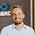Saturday, August 23, 2014

[UE3/UE4] Ocean Waves Normals

Using the world position offset (aka vertex shader) is a popular way of creating large scale ocean waves in unreal engine 3/4. The drawback is that any kind of deformed geometry won't affect the shading at all. That's why you need to manually calculate the new normals inside the material.
To do so it is necessary to calculate the derivatives of the same function which is used to form the ocean waves. The derivatives with respect to x and y are equivalent to the red and green channel of a normal map. Given these 2 components it is possible to calculate the missing 3rd component to get the final per pixel normals.
I'm well aware that there are many ways of implementing ocean motion but I decided to only cover one approach with 2 sub-types, directional waves and circular waves.
In a realistic ocean material you would usually combine multiple waves of different size, speed, direction/position, which also means you would need to duplicate the material graphs shown in this tutorial. Personally I wouldn't calculate the normal for more than 3-4 waves to keep the overall material complexity at a moedrate level.

The material setups below will only work properly if  "Tangent Space Normal" is unchecked in the material properties. This is due to the fact that all calculations are done in world space which also means the generated normals are in world space. If you like to combine these normals with a normal map you would need to do transformation from world to tangent space first.

Directional Waves

Directional waves are based on the dot product between the world position of each pixel and a world space direction vector. This dot product gets added to the time and then fed into a sine node to move the vertices along the z-axis.

Generating normals for directional waves is rather easy. The sine node is being replaced by cosine and then multiplied by the direction vectors' x- and y-component.
In the example below you can also see that the normal intensity is automatically calculated by comparing the length and height of a wave.

Circular Waves

Circular waves are defined by an origin point and the distance between this origin and the world position. Similar to the directional wave setup this distance is added to time and then fed into a sine node.

The material setup for calculating the derivatives for circular waves is a bit more complex, because the distance node involves a square root.

1.Great stuff. Your node formulas produce the best results from the simplest setups out of many that I attempted or copied.

2.Hello. I really appreciate your work and shring it with us. I got to this:

The material setups below will only work properly if "Tangent Space Normal" is unchecked in the material properties.

I can't seem to find it in UE4. can you please tell me where it is (in the IDE I mean).
Thanks a lot.

3.I fount "Tangent Space Normal", but still have an error. I don;t have the same result as you do on directional waves. I put the material on a basic shape cube and it bens only in the middle instead of the position of each wave. Do you have any ideea what the problem might be?